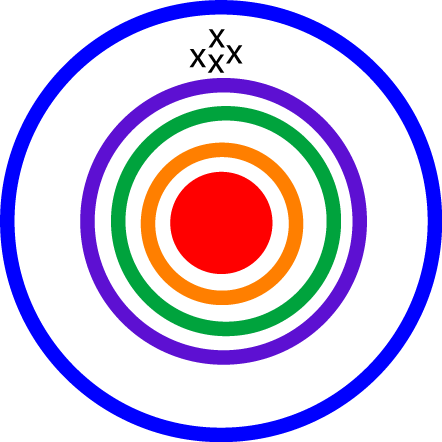# Definition of PrecisionThe precision of a measurement tells you how close two or measurements of the same quantity are to each other.

For example, if you weigh the same object five times and get the same measurement of $1.2 \text{ kg}$ each time, then your measurements are very precise.

However, if you get five different measurements, like $1.2 \text{ kg}$, $1.5 \text{ kg}$, $2.4 \text{ kg}$, $0.7 \text{ kg}$ and $1.1 \text{ kg}$, then your measurements aren't very precise.

### Description

The aim of this dictionary is to provide definitions to common mathematical terms. Students learn a new math skill every week at school, sometimes just before they start a new skill, if they want to look at what a specific term means, this is where this dictionary will become handy and a go-to guide for a student.

### Audience

Year 1 to Year 12 students

### Learning Objectives

Learn common math terms starting with letter P

Author: Subject Coach
You must be logged in as Student to ask a Question.